# Movement of Golf Ball, Impact (Dynamic Mesh), ANSYS Fluent Simulation Training

120.00 \$

In this research, the movement of the golf ball due to the impact force of 200 Newtons with an angle of 30 degrees has been investigated and the movement path of the ball has been determined.

This product includes Geometry & Mesh file and a comprehensive Training Movie.

There are some free products to check our service quality.

To order your ANSYS Fluent project (CFD simulation and training), contact our experts via [email protected], online support, or WhatsApp.

Click on Add To Cart and obtain the Geometry file, Mesh file, and a Comprehensive Training Video.

To Order Your Project or benefit from a CFD consultation, contact our experts via email ([email protected]), online support tab, or WhatsApp at +1 (903) 231-3943.

## Introduction

In dynamic simulation, quasi-experimental aerodynamic coefficients are used. These coefficients are obtained by quasi-experimental software. The coefficients obtained in these softwares have many errors. To achieve more accurate coefficients, numerical simulation by computational fluid dynamics is used and the coefficients obtained are calibrated by semi-experimental simulation software using CFD coefficients. In this research, the movement of the golf ball due to the impact force of 200 Newtons with an angle of 30 degrees has been investigated by ANSYS Fluent software and the movement path of the ball has been determined.

## Problem Description

In this project, a golf ball movement with an aerodynamic point of view has been studied, the force which is applied to the ball is equal to 200 N. The golf ball displacement due to the impact, has been studied in terms of time.

## Golf Ball Geometry & Mesh

We design the present model in three dimensions using CAD software. We carry out the model’s meshing using ICEM software. The mesh type is unstructured. The element number is equal to 945765.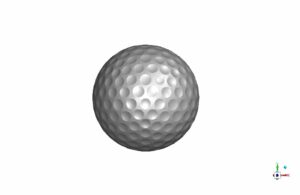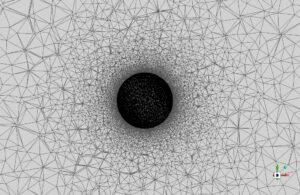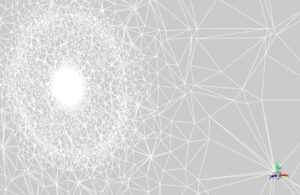## Golf Ball Movement CFD Simulation Strategy

The issue has been investigated numerically using Fluent commercial software. This problem is solved in transient mode using the pressure-based method.

Fluent software is used to solve the governing equations numerically. This chapter considers flow conditions, type of selected boundaries, type of solvent, and flow discretization methods.

### Material Properties

In this research, the air has been used as a fluid. The following table shows the air properties extracted from the Fluent software database, given that the current is in an incompressible regime and its density is constant.

 Amount (units) Fluid Properties(air) 1006.43 (J/kg.K) Specific Heat 0.0242(w/m.k) Thermal conductivity

### Boundary Conditions

One of the most influential variables in the numerical solution process is boundary conditions. For this purpose, we specify different boundary conditions in the computational domain. These conditions are introduced below.
– Wall
– pressure-far-field

 models K-W viscous model SST K-W model Dynamic mesh Active Mesh methods Smoothing & Remeshing Six DOF Active boundary conditions Pressure-far-field inlet 0.0001 Mach number wall wall of body stationary wall wall motion solution methods couple pressure velocity coupling second order pressure spatial discretization second order upwind momentum first order upwind turbulent kinetic energy first order upwind turbulent dissipation rate initialization standard initialization method 0 (Pa) gauge pressure 0.0347 (m/s) x-velocity 0 (m/s) y-velocity , z-velocity

## Solution Convergence

In computational fluid dynamics, we use iterative solutions to arrive at the answer to the problem. These iterative solutions start with the initial values ​​and continue solving until they reach the convergence criteria or the number of steps specified by the user. We define different criteria for the convergence of the problem. One of the most widely used criteria for determining the convergence of a problem is residual values. residuals are the sum of the differences in the values ​​calculated on all cells in the current and previous time steps. They are calculated in each iteration, and the solution continues until its value is less than the criterion specified by the user. We usually recommend to reduce the residual values ​​by 3 or 4 times. As shown in the figure below, all residuals, including velocities and parameters of the perturbation model, are smaller than the standard 10e-3.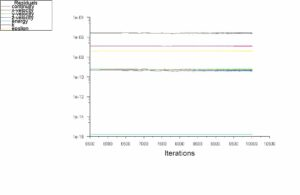## Golf Ball Movement Results & Discussions

In this chapter, we present analyze the simulation results and qualitatively and quantitatively. The quality of the flow around the body has been studied using boundary diagrams.
The following figure shows the location diagrams in the x and y directions in terms of time. We apply a force of 200 Newtons to the golf ball at an angle of 30 degrees to the ground.

F=200 N ,  Fx=173.20  ,  Fy=100.0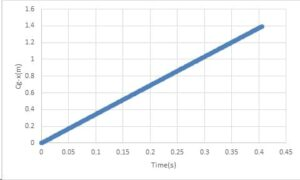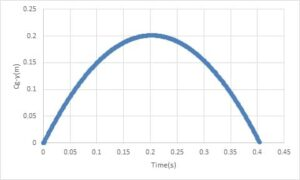We represent the pressure and velocity contours around the golf ball at three different times in the figures.

You can obtain Geometry & Mesh file and a comprehensive Training Movie that presents how to solve the problem and extract all desired results.[/vc_column_text]

## Reviews

There are no reviews yet.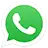Call On WhatsApp